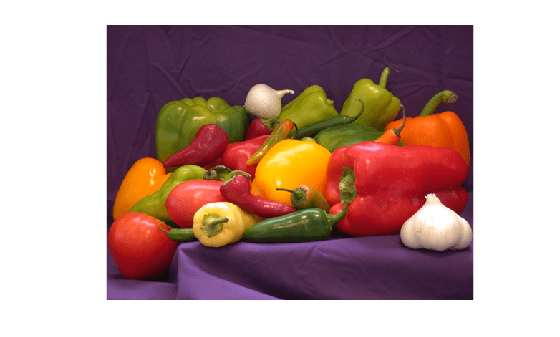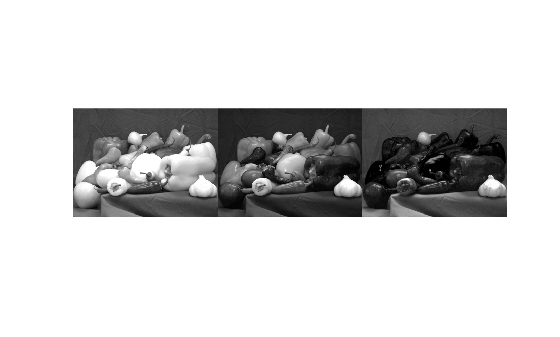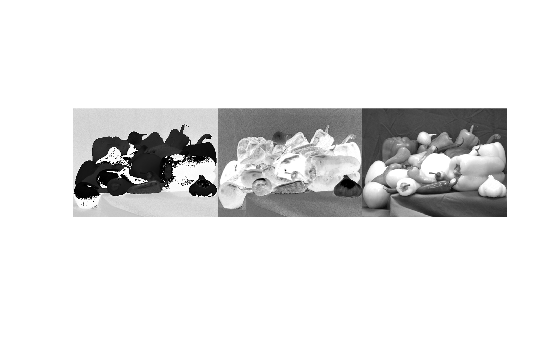# imsplit

Split multichannel image into its individual channels

## Syntax

``[c1,c2,c3,...,ck] = imsplit(I)``

## Description

example

````[c1,c2,c3,...,ck] = imsplit(I)` returns a set of k images representing the individual channels in the k-channel image `I`.```

## Examples

collapse all

Read an RGB image into the workspace and display the image.

```I = imread('peppers.png'); imshow(I)```Split the image into its component red, green, and blue channels.

`[r,g,b] = imsplit(I);`

Display the three color channels as a montage. Red peppers have a signal predominantly in the red channel. Yellow and green peppers have a signal in both the red and green channels. White objects, such as the garlic in the foreground, have a strong signal in all three channels.

`montage({r,g,b},'Size',[1 3])`Read an RGB image into the workspace and display the image.

```rgbImage = imread('peppers.png'); imshow(rgbImage)```Convert the RGB image to the HSV colorspace by using the `rgb2hsv` function.

`hsvImage = rgb2hsv(rgbImage);`

Split the HSV image into its component hue, saturation, and value channels.

`[h,s,v] = imsplit(hsvImage);`

Display the three channels as a montage.

`montage({h,s,v},'Size',[1 3])`## Input Arguments

collapse all

Input image, specified as an m-by-n-by-k numeric array.

Data Types: `single` | `double` | `int8` | `int16` | `int32` | `int64` | `uint8` | `uint16` | `uint32` | `uint64` | `logical`

## Output Arguments

collapse all

Output images, returned as k individual numeric matrices, where k is the number of channels in the input image. The output images are the same class as the input image.

## Version History

Introduced in R2018b